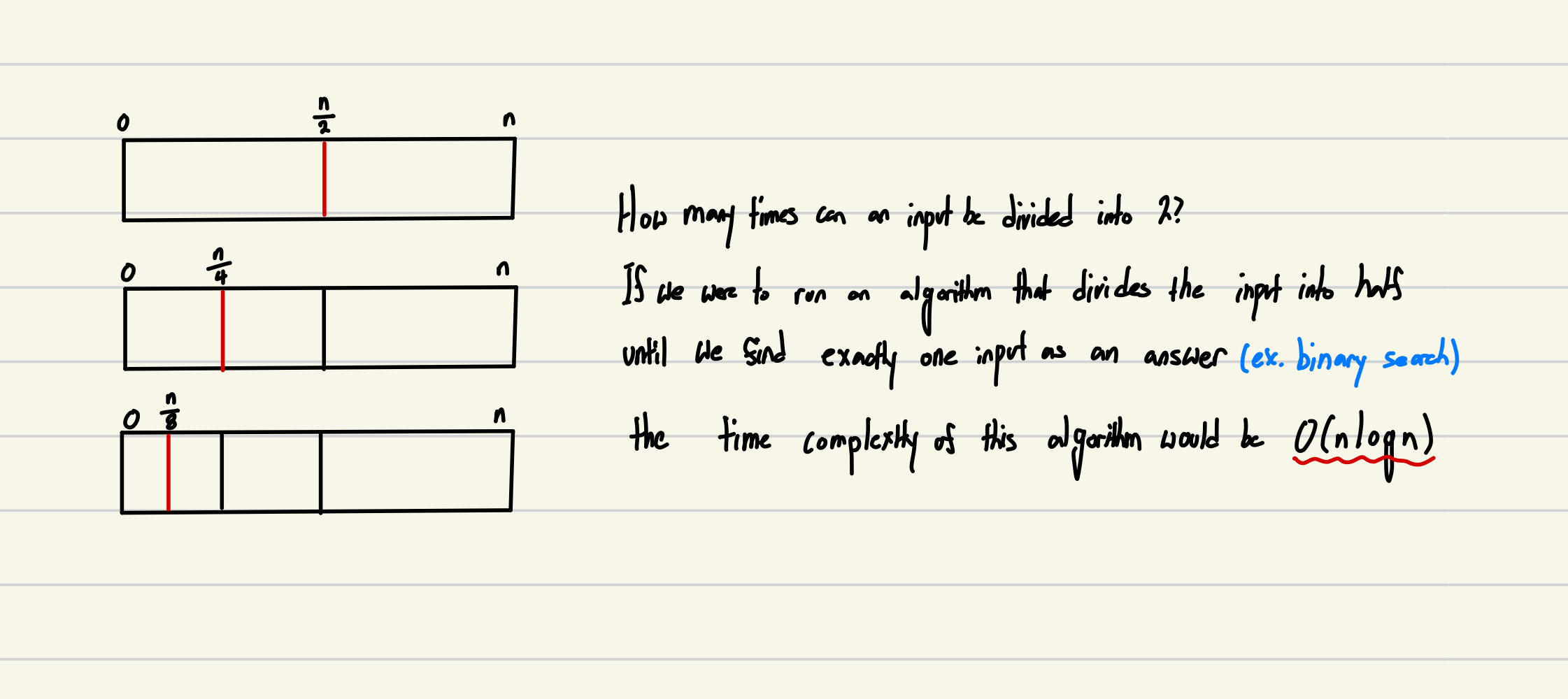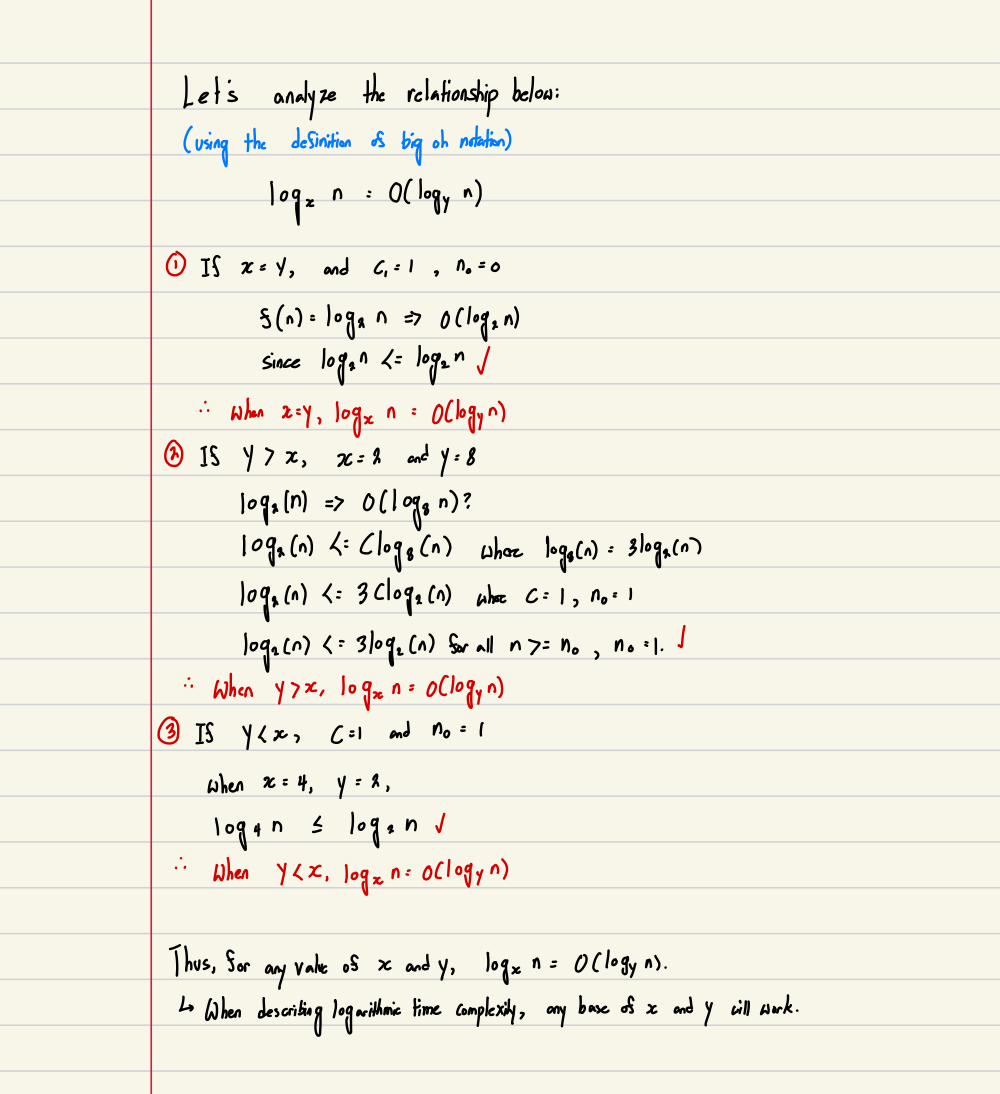# Logarithmic Time Complexity

choikh0423·2022년 5월 11일
0

## Time Complexity

목록 보기
1/1## What is logarithms?

Logarithm basically asks how many times I would have to multiply the base to get the target number.
As a simple example, log(1000) means 10^x = 1000, where x becomes 3.

## How is this used in Computer Science?

In Computer Science, logarithms play a huge role in analyzing algorithms that only evaluates half of its input.## What if we are not dividing the input into just half?

If we decide to divide the input by 1/3 or 1/4 or any other fraction, the base of log will be different.

But... Would it matter?
Let's try anlayzing this refering back to the definition of Big Oh Notation.
Refer to the document on Big Oh Notation if you are not familiar with the concept.In conclusion, we can neglect whatever base the logarithm has when describing time complexity in Big Oh Notation.